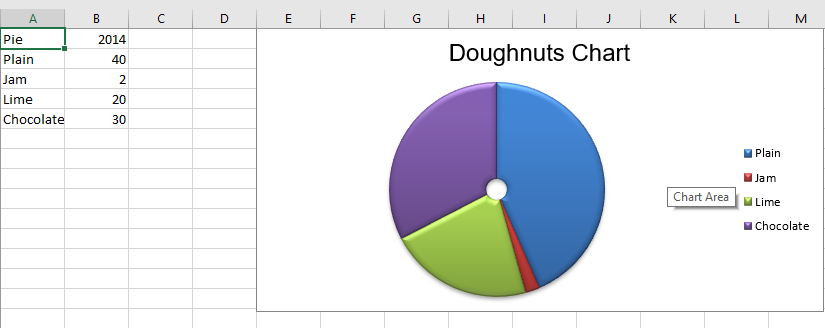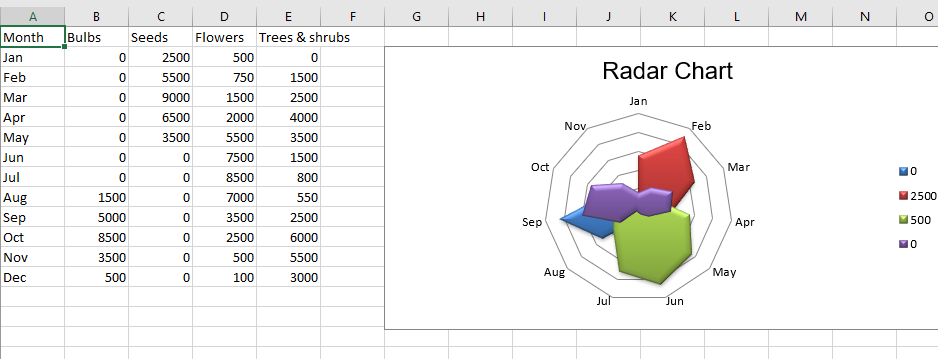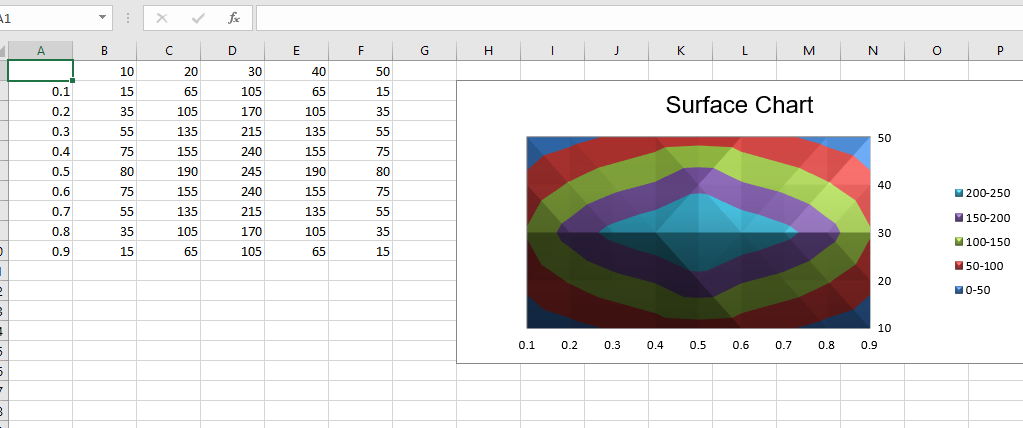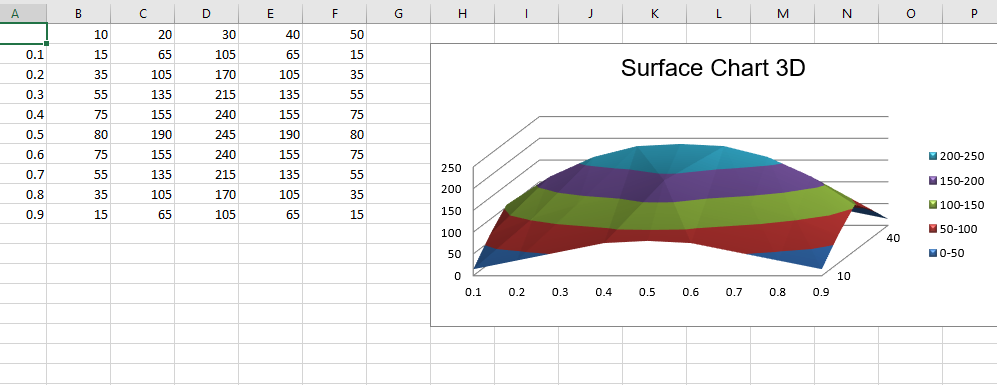Python | Plotting charts in excel sheet using openpyxl module | Set 3

• Difficulty Level : Hard
• Last Updated : 19 May, 2021

Prerequisite : Plotting charts in excel sheet using openpyxl module Set – 1 | Set – 2
Openpyxl is a Python library using which one can perform multiple operations on excel files like reading, writing, arithmetic operations and plotting graphs.
Charts are composed of at least one series of one or more data points. Series themselves are comprised of references to cell ranges. Let’s see how to plot Doughnot, Radar, Surface, 3D Surface Chart on an excel sheet using openpyxl.
For plotting the charts on an excel sheet, firstly, create chart object of specific chart class( i.e SurfaceChart, RadarChart etc.). After creating chart objects, insert data in it and lastly, add that chart object in the sheet object. Let’s see how to plot different charts using realtime data.
Code #1 : Plot the Doughnut Chart
Doughnut charts are similar to pie charts except that they use a ring instead of a circle. They can also plot several series of data as concentric rings. For plotting the Doughnut chart on an excel sheet, use DoughnutChart class from openpyxl.chart submodule.

Python3

 # import Workbook from openpyxlfrom openpyxl import Workbook # import DoughnutChart, Reference from openpyxl.chart sub_module .from openpyxl.chart import DoughnutChart, Reference # import DataPoint from openpyxl.chart.series classfrom openpyxl.chart.series import DataPoint # Call a Workbook() function of openpyxl# to create a new blank Workbook objectwb = Workbook() # Get workbook active sheet# from the active attribute.ws = wb.active # data givendata = [    ['Pie', 2014],    ['Plain', 40],    ['Jam', 2],    ['Lime', 20],    ['Chocolate', 30],] # write content of each row in 1st and 2nd# column of the active sheet respectively .for row in data:    ws.append(row) # Create object of DoughnutChart classchart = DoughnutChart() # create data for plottinglabels = Reference(ws, min_col = 1, min_row = 2, max_row = 5)data = Reference(ws, min_col = 2, min_row = 1, max_row = 5) # adding data to the Doughnut chart objectchart.add_data(data, titles_from_data = True) # set labels in the chart objectchart.set_categories(labels) # set the title of the chartchart.title = "Doughnuts Chart" # set style of the chartchart.style = 26 # add chart to the sheet# the top-left corner of a chart# is anchored to cell E1 .ws.add_chart(chart, "E1") # save the filewb.save("doughnut.xlsx")

Output:Code #2: Plot the Radar Chart
Data that is arranged in columns or rows on a worksheet can be plotted in a radar chart. Radar charts compare the aggregate values of multiple data series. It is effectively a projection of an area chart on a circular x-axis. For plotting the Radar chart on an excel sheet, use RadarChart class from openpyxl.chart submodule.

Python3

Output:Code #3 : Plot The Surface Chart
Data that is arranged in columns or rows on a worksheet can be plotted in a surface chart. A surface chart is useful when you want to find optimum combinations between two sets of data. As in a topographic map, colors and patterns indicate areas that are in the same range of values. For plotting the Surface chart on an excel sheet, use SurfaceChart class from openpyxl.chart submodule.

Python3

 # import Workbook from openpyxlfrom openpyxl import Workbook # import SurfaceChart, Reference, Series from openpyxl.chart sub_module .from openpyxl.chart import SurfaceChart, Reference, Series # Call a Workbook() function of openpyxl# to create a new blank Workbook objectwb = Workbook() # Get workbook active sheet# from the active attribute.ws = wb.active # given datadata = [    [None, 10, 20, 30, 40, 50, ],    [0.1, 15, 65, 105, 65, 15, ],    [0.2, 35, 105, 170, 105, 35, ],    [0.3, 55, 135, 215, 135, 55, ],    [0.4, 75, 155, 240, 155, 75, ],    [0.5, 80, 190, 245, 190, 80, ],    [0.6, 75, 155, 240, 155, 75, ],    [0.7, 55, 135, 215, 135, 55, ],    [0.8, 35, 105, 170, 105, 35, ],    [0.9, 15, 65, 105, 65, 15],] # write content of each row in 1st and 2nd# column of the active sheet respectively .for row in data:    ws.append(row) # Create object of SurfaceChart classchart = SurfaceChart() # create data for plottinglabels = Reference(ws, min_col = 1, min_row = 2, max_row = 10)data = Reference(ws, min_col = 2, max_col = 6, min_row = 1, max_row = 10) # adding data to the Surface chart objectchart.add_data(data, titles_from_data = True) # set labels in the chart objectchart.set_categories(labels) # set the title of the chartchart.title = "Surface Chart" # set style of the chartchart.style = 26 # add chart to the sheet# the top-left corner of a chart# is anchored to cell H2 .ws.add_chart(chart, "H2") # save the filewb.save("Surface.xlsx")

Output:Code #4 : Plot the Surface 3D chart
For plotting the 3D Surface chart on an excel sheet, use SurfaceChart3D class from openpyxl.chart submodule.

Python3

 # import Workbook from openpyxlfrom openpyxl import Workbook # import SurfaceChart3D, Reference, Series from openpyxl.chart sub_module .from openpyxl.chart import SurfaceChart3D, Reference, Series # Call a Workbook() function of openpyxl# to create a new blank Workbook objectwb = Workbook() # Get workbook active sheet# from the active attribute.ws = wb.active # given datadata = [    [None, 10, 20, 30, 40, 50, ],    [0.1, 15, 65, 105, 65, 15, ],    [0.2, 35, 105, 170, 105, 35, ],    [0.3, 55, 135, 215, 135, 55, ],    [0.4, 75, 155, 240, 155, 75, ],    [0.5, 80, 190, 245, 190, 80, ],    [0.6, 75, 155, 240, 155, 75, ],    [0.7, 55, 135, 215, 135, 55, ],    [0.8, 35, 105, 170, 105, 35, ],    [0.9, 15, 65, 105, 65, 15],] # write content of each row in 1st and 2nd# column of the active sheet respectively .for row in data:    ws.append(row) # Create object of SurfaceChart3D classchart = SurfaceChart3D() # create data for plottinglabels = Reference(ws, min_col = 1, min_row = 2, max_row = 10)data = Reference(ws, min_col = 2, max_col = 6, min_row = 1, max_row = 10) # adding data to the Surface chart 3D objectchart.add_data(data, titles_from_data = True) # set labels in the chart objectchart.set_categories(labels) # set the title of the chartchart.title = "Surface Chart 3D" # set style of the chartchart.style = 26 # add chart to the sheet# the top-left corner of a chart# is anchored to cell H2 .ws.add_chart(chart, "H2") # save the filewb.save("Surface3D.xlsx")

Output :My Personal Notes arrow_drop_up